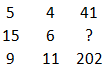Question 18

# Study the given pattern carefully and select the number that can replace the question mark (?) in it.Solution

$$5^2 + 4^2$$ = 25 + 16 = 41
$$9^2 + 11^2$$ = 81 + 121 = 202
Similarly,
$$(15)^2 + 6^2$$ = 225 + 36 = 261
$$\therefore$$ The correct answer is option C.

• Free SSC Study Material - 18000 Questions
• 230+ SSC previous papers with solutions PDF

##### Subhojit Roy

3 years, 5 months ago

Solution?

OR